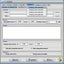# MathProf for Windows

## Author's review

MathProf is an easy to use mathematics program. Through the use of two and three dimensional graphics, MathProf can display mathematical correlations in a very clear and simple way.

The program covers the following areas in approximately 180 subroutines:

Analysis
Geometry
Algebra
3D Mathematics
Stochastics
Vector algebra
Linear algebra

MathProf helps Junior High School students with problems in Geometry and Algebra. High School and College students, seeking to expand their knowledge into further reaching mathematical concepts find this program very useful as well.